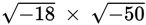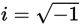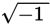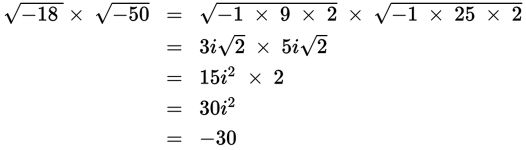# SAT Math Multiple Choice Question 947: Answer and Explanation

### Test Information

Question: 947

15. What iswritten in simplest form? (Note:)

• A. -30
• B. -30i
• C. 30
• D. 30i

Explanation:

A

Difficulty: Medium

Category: Additional Topics in Math / Imaginary Numbers

Strategic Advice: You must be very careful when multiplying square roots of negative numbers. You cannot simply multiply the two negative signs together. Instead, write each factor as an imaginary number, and then multiply the results together.

Getting to the Answer: Write the number under each radical as a product of -1 and convenient factors of the number. Then you can move an i to the outside of the radical (becauseis defined as i):